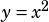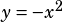y = x^2 + a
y = -x^2 + b

In the system of equations above, a and b are constants. Which of the following must be true if th esystem of equations has two solutions?

A) a is less than b.
B) a is greater than b.
C) a is equal to b.
D) a and b are both equal to zero.

If you know your function translations, you know that when a constant is added to a function like, that constant shifts the function vertically up (if the constant is positive) or down (if the constant is negative). Because we know thatis a standard parabola facing up andis a parabola facing down, we can use our knowledge of vertical shifts to solve this.The functions will only intersect twice if the vertex of the downward facing one is higher than the vertex of the upward facing one, as shown in the figure above. Therefore, a must be less than b.

For proof, try a few examples in your calculator. Below I’ve set a to 2 and b to 5. Looks good!